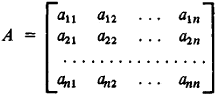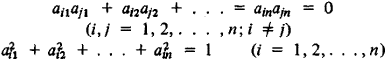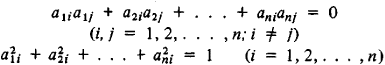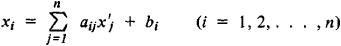# Orthogonal Matrix

(redirected from Special orthogonal matrix)
Also found in: Dictionary.

## orthogonal matrix

[ȯr′thäg·ən·əl ′mā·triks]
(mathematics)
A matrix whose inverse and transpose are identical.

## Orthogonal Matrix

An orthogonal matrix of order n is a matrixwhose product with the transpose A′ gives the identity matrix, that is, AA′ = E and AA = E. The elements of an orthogonal matrix satisfy the relationsor the equivalent relationsThe determinant ǀAǀ of an orthogonal matrix is equal to +1 or – 1. The product of two orthogonal matrices is an orthogonal matrix. All orthogonal matrices of order n form, with respect to the operation of multiplication, a group called the orthogonal group. In conversion from one rectangular coordinate system to another, the coefficients aij in the coordinate transformation equationsform an orthogonal matrix.

Site: Follow: Share:
Open / Close